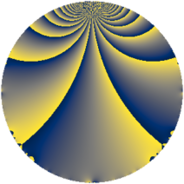# Properties

 Label 152.1.uLevel $152$ Weight $1$ Character orbit 152.u Rep. character $\chi_{152}(35,\cdot)$ Character field $\Q(\zeta_{18})$ Dimension $6$ Newform subspaces $1$ Sturm bound $20$ Trace bound $0$

# Related objects

## Defining parameters

 Level: $$N$$ $$=$$ $$152 = 2^{3} \cdot 19$$ Weight: $$k$$ $$=$$ $$1$$ Character orbit: $$[\chi]$$ $$=$$ 152.u (of order $$18$$ and degree $$6$$) Character conductor: $$\operatorname{cond}(\chi)$$ $$=$$ $$152$$ Character field: $$\Q(\zeta_{18})$$ Newform subspaces: $$1$$ Sturm bound: $$20$$ Trace bound: $$0$$

## Dimensions

The following table gives the dimensions of various subspaces of $$M_{1}(152, [\chi])$$.

Total New Old
Modular forms 18 18 0
Cusp forms 6 6 0
Eisenstein series 12 12 0

The following table gives the dimensions of subspaces with specified projective image type.

$$D_n$$ $$A_4$$ $$S_4$$ $$A_5$$
Dimension 6 0 0 0

## Trace form

 $$6 q - 3 q^{3} - 3 q^{6} - 3 q^{8} - 3 q^{9} + O(q^{10})$$ $$6 q - 3 q^{3} - 3 q^{6} - 3 q^{8} - 3 q^{9} + 6 q^{18} + 6 q^{22} - 3 q^{24} + 3 q^{27} - 3 q^{33} - 3 q^{36} - 3 q^{38} - 3 q^{41} + 6 q^{44} + 6 q^{48} - 3 q^{49} - 3 q^{50} + 3 q^{51} - 3 q^{54} - 3 q^{59} - 3 q^{64} - 3 q^{66} - 3 q^{67} + 3 q^{68} + 6 q^{72} + 6 q^{73} + 3 q^{81} - 3 q^{82} - 3 q^{97} + 3 q^{99} + O(q^{100})$$

## Decomposition of $$S_{1}^{\mathrm{new}}(152, [\chi])$$ into newform subspaces

Label Dim $A$ Field Image CM RM Traces $q$-expansion
$a_{2}$ $a_{3}$ $a_{5}$ $a_{7}$
152.1.u.a $6$ $0.076$ $$\Q(\zeta_{18})$$ $D_{9}$ $$\Q(\sqrt{-2})$$ None $$0$$ $$-3$$ $$0$$ $$0$$ $$q-\zeta_{18}^{5}q^{2}+(\zeta_{18}^{6}-\zeta_{18}^{7})q^{3}-\zeta_{18}q^{4}+\cdots$$